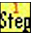Roman Numerals Explained
Stephen P. Morse, San Francisco

Roman Numerals are a way of encoding numbers using seven letters in the Latin alphabet. The seven letters and their associated numeric values are as follows:
I = 1
V = 5
X = 10
L = 50
C = 100
D = 500
M = 1000
There are very specific rules for how the letters are selected. Specifically:
1. Starting with the highest valued letter (M), keep repeating that letter as long as the sum of all the letters does not exceed the numeric value desired.
2.Repeat step 1 for each lower-valued letter in turn.
3. Four repetitions of the same letter are not allowed. So make the following replacements (these are referred to as subtractive notation)
IIII not preceded by V => IV
VIIII => IX
XXXX not preceded by L => XL
LXXXX => XC
CCCC not preceded by D => CD
DCCCC => CM
MMMM is not permitted (the highest possible number is 3999)
4. Exception: The number 4 on clocks is sometimes written as IIII instead of IV.
Examples:
III = 3
IV = 4
IX = 9
XXV = 25
XCIX = 99
MCMLXXXIV = 1984
Large Numbers:
1. Large numbers are sometimes written with a bar over them (Vinculum notation).
For example, V̅=5000, X̅=10000, L̅=50000, C̅=100000, etc.

2. Large numbers are sometimes written with archaic symbols.
For example, ↁ=5000, ↂ=10000, ↇ=50000, ↈ=100000, etc.

3. A modern version of the archaic symbols is the Apostrophus notation.

 500 1,000 5,000 10,000 50,000 100,000 500,000 1,000,000 Vinculum D M V̅ X̅ L̅ C̅ D̅ M̅ Archaic D ↀ ↁ ↂ ↇ ↈ Apostrophus |Ↄ C|Ↄ |ↃↃ CC|ↃↃ |ↃↃↃ CCC|ↃↃↃ |ↃↃↃↃ CCCC|ↃↃↃↃ